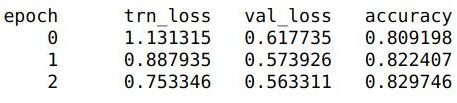# Overfitting on dog breeds classification[SOLVED]

I use resnet34 with data augmentation. Firstly I train it with frozen weights then unfreeze weights and continue training with differential learning rates. Why does it start t overfit so much after unfreezing weights? Am I doing something wrong?

``````arch =     resnet34
PATH = 'data/dog_breeds/'
sz = 224

n = len(list(open(f'{PATH}labels.csv'))) - 1
val_idxs = get_cv_idxs(n)

def get_data(sz):
tfms = tfms_from_model(arch, sz, aug_tfms=transforms_side_on, max_zoom=1.1)
data = ImageClassifierData.from_csv(PATH, 'train', f'{PATH}labels.csv', bs=32, tfms=tfms,
val_idxs=val_idxs, suffix='.jpg', test_name='test', num_workers=3)
return data

data = get_data(sz)
learn = ConvLearner.pretrained(resnet34, data)

learn.lr_find()
``````

``````lrs = [1e-4, 1e-3, 5e-2]
lr = 5e-2

learn.fit(lr, 3, cycle_len=1)
````````````learn.unfreeze()
learn.fit(lrs, 2, cycle_len=2, cycle_mult=2)
``````(After last epoch training loss is a lot smaller than validation one, it wasn’t the case for frozen model)

The reason for overfitting were the high learning rates for first resnet layers. Decreasing them helped me to get a lot better results.

2 Likes

I’m reopening this because I’ve seen the same.

But why does overfitting occure if we use a learning rate that is “too high”?
e.g. I tried differential learning rates of `[1e-5,1e-3,1e-2]` instead of the “normal” `[1e-4,1e-3,1e-2]` and got a accuracy improvement of about 1%. What is the intuition behind it?

Intuitively: The first few layers are already well trained (pretrained) to recognize abstract features. Yet if you allow them to be trained with too high a learning rate, they will home in on the specific things in your train set, and that’s not what we want; the first few layers should still just keep recognizing abstract high level features (edges, corners, circles, and then eyes, noses, …). The main learning happens in the final few layers where we’re putting it all together to tell one dog from another dog.